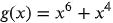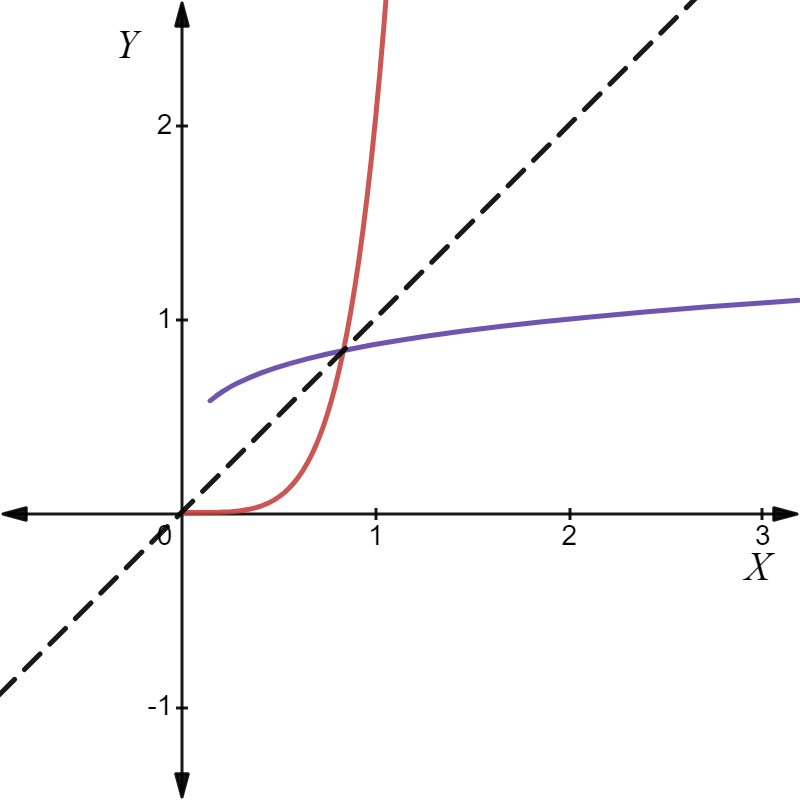1
56
views
58b
Problem

Problem 58b

Chapter 1: Functions and ModelsTextbook ExpertVerified Tutor
14 Oct 2021

Given information

The given function areand.

Step-by-step explanation

Step 1.

Use the graphing calculator to graph the given function.

Red color line denotes the functionBlue color line denotes the function